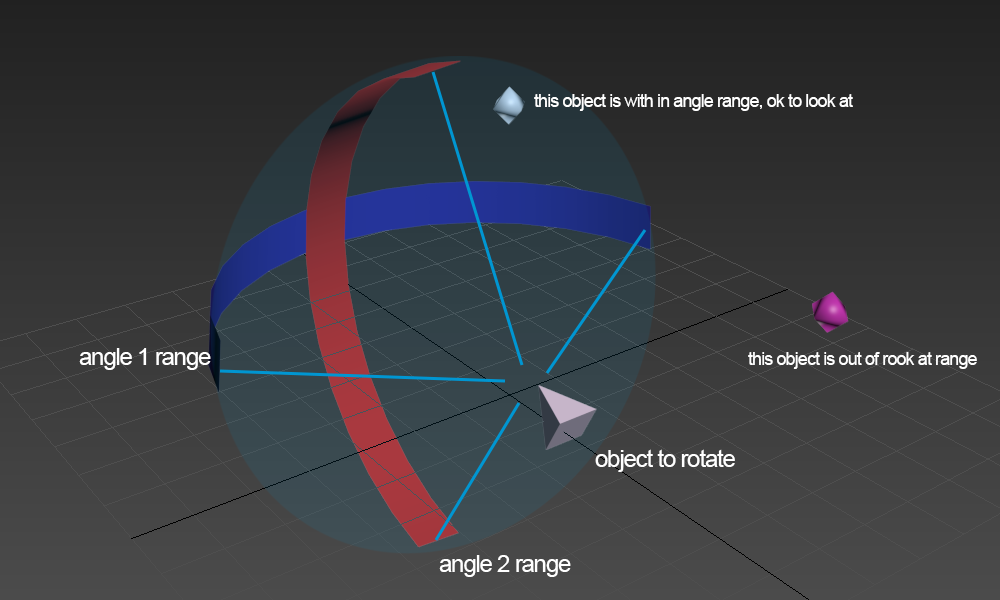Jump to content

# lookat clamp on both Axes

## Recommended Posts

Trying to do a lookat clamp on both axes, the attached image shows my problem.

my goal is to set a mesh to look at some object only if both axes are fall within clamp range.

I found similar thread, but only discuss on one axis only.

Very appreciated if anyone could provide direction or solution on this problem, thanks~##### Share on other sites

Simplest solution: attach an invisible mesh (sphere or box) that fits your "range/shape" to your "object to rotate" and check .intesectsMesh(objectToLookAt).

##### Share on other sites

Thanks @max123 for simple solution, I am also trying to solve it mathematically, might try your method later if mine is not working.

I guess the problem can be solved with some clamp and calculation in spherical coordinate space.

After watching https://www.youtube.com/watch?v=-ny7psXNdUU , I was able to covert Cartesian to spherical space

here is the conversion I came up so far

I think it is similar to how ArchRotateCamera moves, will try later for clamping functions, very appreciated for any other suggestions

##### Share on other sites

Hi amo,
look, this is the source to lookAt() ...

``````public lookAt(targetPoint: Vector3, yawCor: number = 0, pitchCor: number = 0, rollCor: number = 0, space: Space = Space.LOCAL): void {
/// <summary>Orients a mesh towards a target point. Mesh must be drawn facing user.</summary>
/// <param name="targetPoint" type="Vector3">The position (must be in same space as current mesh) to look at</param>
/// <param name="yawCor" type="Number">optional yaw (y-axis) correction in radians</param>
/// <param name="pitchCor" type="Number">optional pitch (x-axis) correction in radians</param>
/// <param name="rollCor" type="Number">optional roll (z-axis) correction in radians</param>
/// <returns>Mesh oriented towards targetMesh</returns>

var dv = AbstractMesh._lookAtVectorCache;
var pos = space === Space.LOCAL ? this.position : this.getAbsolutePosition();
targetPoint.subtractToRef(pos, dv);
var yaw = -Math.atan2(dv.z, dv.x) - Math.PI / 2;
var len = Math.sqrt(dv.x * dv.x + dv.z * dv.z);
var pitch = Math.atan2(dv.y, len);
this.rotationQuaternion = this.rotationQuaternion || new Quaternion();
Quaternion.RotationYawPitchRollToRef(yaw + yawCor, pitch + pitchCor, rollCor, this.rotationQuaternion);
}
``````

So, i would make my own lookAt function and add the clamp function just before the QuaternionRotaion.

##### Share on other sites

Hi,
i just ran in a very similar problem. Maybe this can help.

``````targetFrontality( target ){
//get the directional vector from viewer to target
var d = viewer.position.subtract( target.position );
d.normalize();

//generate a heading vector for the viewer, here the viewer looks along the z axis in positive direction
var v = new BABYLON.Vector3(0,0,1);

//now calculate the directional vector of the viewer from the rotation of the viewer
var c = QuaternionMultiplyVector3(viewer._rotationQuaternion, v);
c.normalize();

// the dot product projects one vector on another
// 1.0 = heading strait on
// -1.0 = heading strait away
return BABYLON.Vector3.Dot(d, c);
}

QuaternionMultiplyVector3 (quat,vec) {
var num = quat.x * 2;
var num2 = quat.y * 2;
var num3 = quat.z * 2;
var num4 = quat.x * num;
var num5 = quat.y * num2;
var num6 = quat.z * num3;
var num7 = quat.x * num2;
var num8 = quat.x * num3;
var num9 = quat.y * num3;
var num10 = quat.w * num;
var num11 = quat.w * num2;
var num12 = quat.w * num3;
var result = new BABYLON.Vector3(0,0,0);
result.x = (1 - (num5 + num6)) * vec.x + (num7 - num12) * vec.y + (num8 + num11) * vec.z;
result.y = (num7 + num12) * vec.x + (1 - (num4 + num6)) * vec.y + (num9 - num10) * vec.z;
result.z = (num8 - num11) * vec.x + (num9 + num10) * vec.y + (1 - (num4 + num5)) * vec.z;
return result;
}
``````

if you restrict d and c on axis you can calculate the compontents

##### Share on other sites

After some testing I was able to come up the solution from lookat function from the babylon source as @Klaas suggested, really appreciated for the help.here is a demo

I still don't know what 's the default direction the object is facing without making any rotations.

The demo above I used an empty mesh coneHolder to look at the moving box, The calculated yaw value is between 90 ~ -270 , which I thought was odd (I thought it would be -180 ~ 180 or 0 ~ 360) ,  and pitch value seems to be fine ( -90 ~ 90)

any information or corrections on these ? Thanks for the help  ~

##### Share on other sites
1 hour ago, amo yeh said:

The demo above I used an empty mesh coneHolder to look at the moving box, The calculated yaw value is between 90 ~ -270

This confused me too.

##### Share on other sites

Hi!

Well that looks as if its working nicley. The somewhat wierd degree value of yaw come from this line ..

``var yaw = -Math.atan2(dv.z, dv.x) - Math.PI / 2;``

at the end (-Math.PI / 2) the yaw value is turnt by 90 degree clockwise. At this point you have to insert the yaw offset of the viewer. Wich is the default direction of the facing.

## Join the conversation

You can post now and register later. If you have an account, sign in now to post with your account.
Note: Your post will require moderator approval before it will be visible.Reply to this topic...

×   Pasted as rich text.   Paste as plain text instead

Only 75 emoji are allowed.

×   Your link has been automatically embedded.   Display as a link instead

×   Your previous content has been restored.   Clear editor

×   You cannot paste images directly. Upload or insert images from URL.

Loading...
×
• ### Recently Browsing   0 members

No registered users viewing this page.

×

• #### Activity

• Leaderboard
×
• Create New...